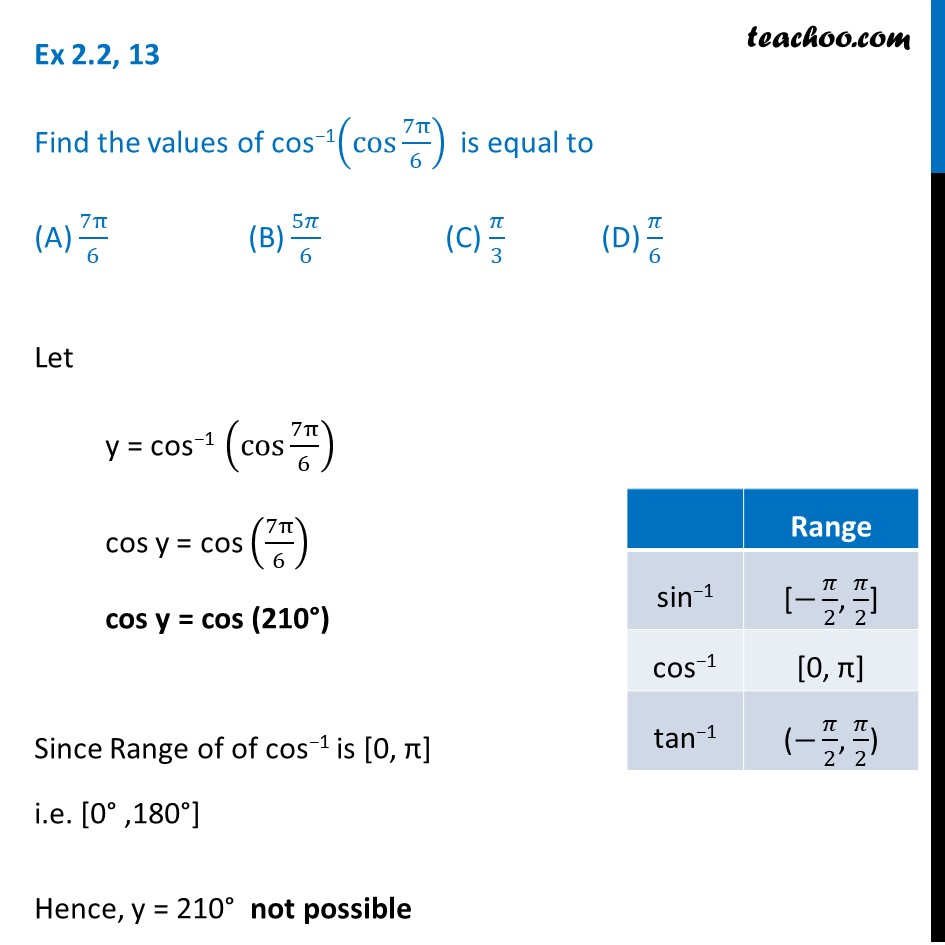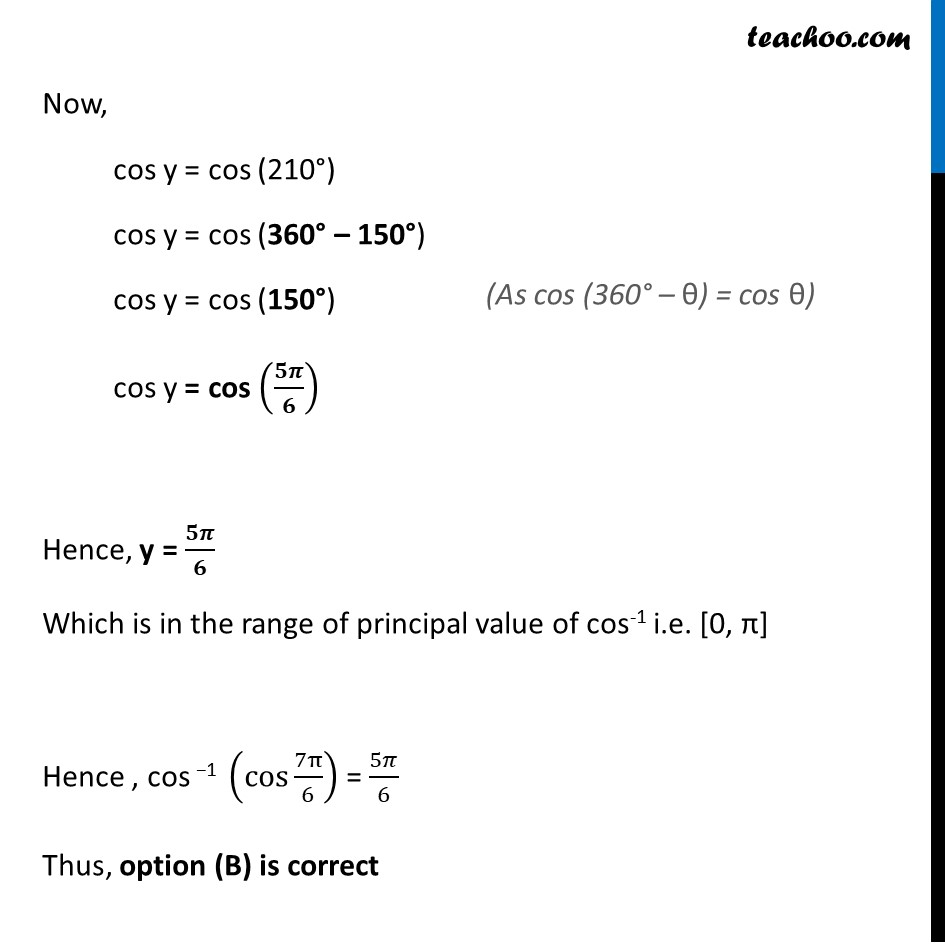Finding principal value

Chapter 2 Class 12 Inverse Trigonometric Functions
Concept wiseLearn in your speed, with individual attention - Teachoo Maths 1-on-1 Class

### Transcript

Ex 2.2, 13 Find the values of cos−1(cos 7π/6) is equal to (A) 7π/6 (B) 5𝜋/6 (C) 𝜋/3 (D) 𝜋/6 Let y = cos−1 (cos 7π/6) cos y = cos (7π/6) cos y = cos (210°) Since Range of of cos−1 is [0, π] i.e. [0° ,180°] Hence, y = 210° not possible Now, cos y = cos (210°) cos y = cos (360° – 150°) cos y = cos (150°) cos y = cos (𝟓𝝅/𝟔) Hence, y = 𝟓𝝅/𝟔 Which is in the range of principal value of cos-1 i.e. [0, π] Hence , cos −1 (cos 7π/6) = 5𝜋/6 Thus, option (B) is correct